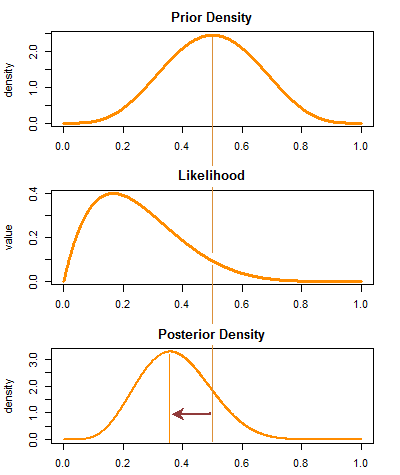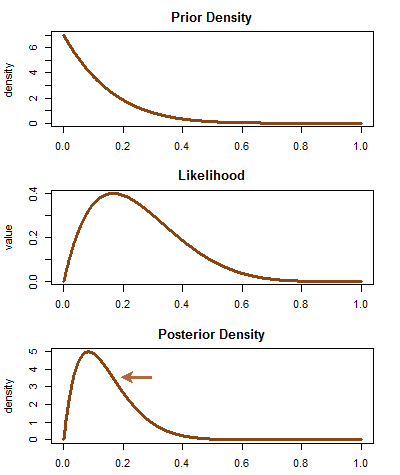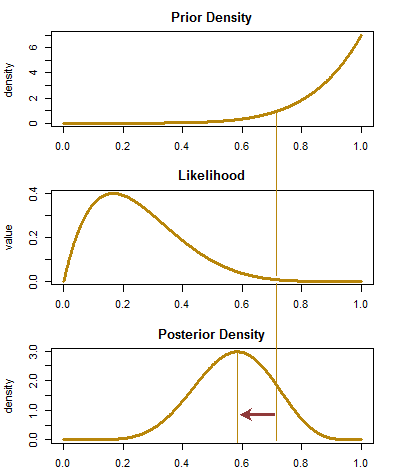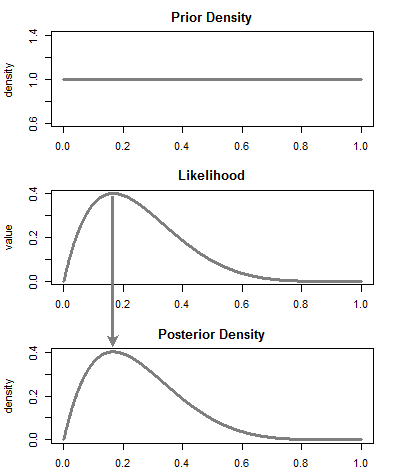### BAYESIAN APPROACH TO ASSESS COIN FAIRNESS:

I’d like to post some illustrations about the way our preconceptions about the “fairness” of the coin (or the experiment in general) affects the posterior probability density, i.e. the $$p\,(\theta\,|\,\text{Data})$$, where $$\theta$$ stands for the probability of ending up with $$\text{heads}$$ in the coin toss.

Luckily, we have a conjugate prior distribution for the binomial case that occupies us - the $$\text{beta}$$ distribution, facilitating the calculation of the posterior distribution.

A prior is conjugate to the likelihood when the posterior is in the same family of distributions as the prior: $$\text{posterior}\, \Pr(\theta|y) \propto \,\text{likelihood}\,\Pr(y|\theta)\,\times\,\text{prior}\,\Pr(\theta)$$. $$y$$ stands for the data observed; $$\theta$$ is the parameter we want to estimate. The binomial-beta is a conjugate model. Let’s call the probability of the binomial $$\theta$$:

\begin{align} p(\theta|y) \propto p(y|\theta)\,p(\theta) &=\text{Bin}(n,\theta) \times \text{Beta}(a,b)\\[2ex] &={n\choose y}\,\theta^y\,(1\,-\,theta)^{(n-y)}\,\times\,\frac{\Gamma(a+b)}{ \Gamma(a)\Gamma(b)}\,\theta^{(a-1)}\,(1-\theta)^{b-1} \propto \theta^y\,(1-\theta)^{(n-y)}\theta^{a-1}(1-\theta)^{b-1}\\[2ex] &=p(\theta|y) \propto \theta^{(y+a-1)}\,(1\,-\,\theta)^{(n-y+b-1)} \end{align}

Hence, the posterior distribution is a $$\text{Beta}\,(y+a,\,n-y+b)$$.

First scenario - The Fair-Minded Player:

We walk into the game (not a very exciting game, but still…), and we have absolutely no reason to assume that there is foul play going on. Things being by nature less than perfect, we have it in our mind that the coin is fair-ish. In other words, we think that the probability of heads, $$\theta$$, falls somewhere around $$\frac{1}{2}$$. Later, the unexpected single $$\text{tail}$$ out of $$6$$ tosses, will force us to move the posterior probability of $$\theta$$ to the left (the arrows indicate the influence of the data on the prior distribution):Second Scenario - The Shrewd Player:

We strongly suspect from insider’s leaked information that the game is markedly biased towards tails, and we not only are about to make a killing, but also in need to further reinforce our conviction after the first round, doubling down our bet:Third Scenario - Losing Your Shirt:

We’ve never played before, but we have read a manual, and we feel ready. All signs clearly indicate that the coin is markedly biased towards $$\text{heads}$$, a mistake that we will soon start to correct at a high $$\$$ cost:Fourth Scenario - No Idea Whatsoever:

It’s a good thing that the $$\text{Beta}(1,1)$$ distribution turns into a $$\text{Unif}\,(0,1)$$ to address this scenario, where only the $$\text{likelihood}$$ will influence the $$\text{posterior}$$ probability of $$\theta$$. As brought up to my attention, a Jeffreys prior is close and possibly more correct:So I hope this provides a bit of a light-hearted visual depiction of our approach to estimating the chances of this game being rigged, perhaps encapsulating more of a real scenario than calculations of the type pbinom(1, 6, 0.5). If you want the code in R, and the credits to a great video with Matlab illustrations, I posted it here.